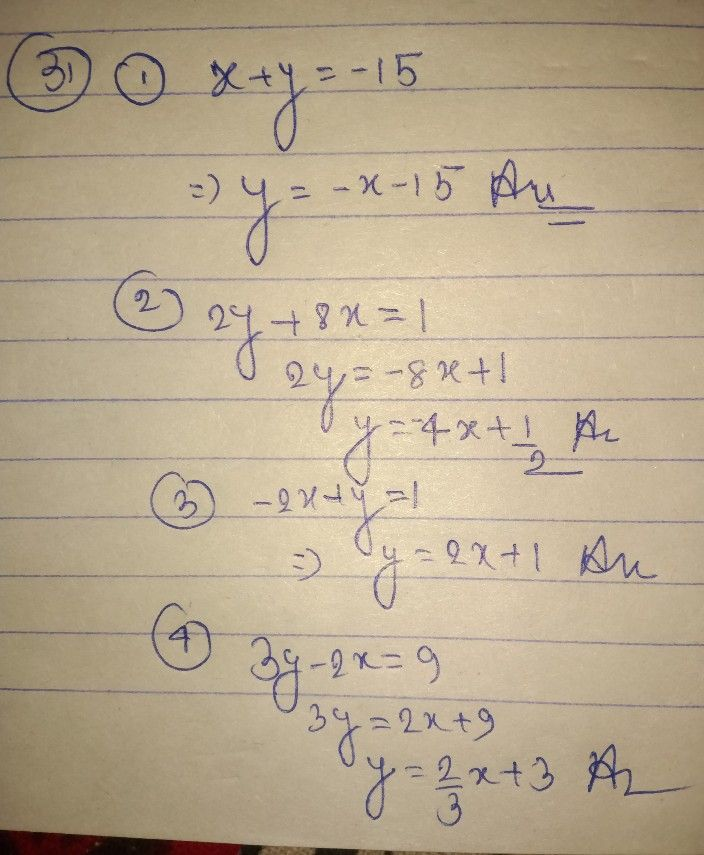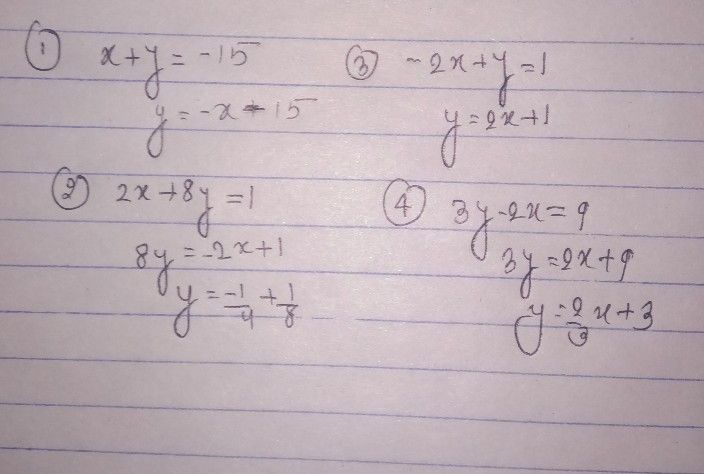Symbol
ProblemRewrite each of the following equation in $y=mx+btorm$ Show each step. $1.x+y=-15$ $2.2y+8x=1$ $3$ $-2x+y=1$ $4.3y-2x=9$
Other
Question content
SolutionQanda teacher - AyushStudent
thanksQanda teacher - Ayush